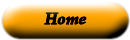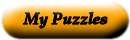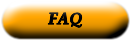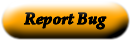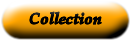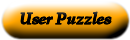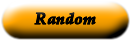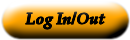### Grade 4 Math Power Words

Match the term to the correct definition.

 product a unit of measure for angles based on dividing up a circle into 360 parts. A unit of measure for temperature. hundredth a letter or other symbol that represents a number example 5 + X = 9 and x=4 to make the number sentence true intersect a number that is written using both a whole number and a fraction right triangle the answer in multiplication acute angle one of two numbers that are multiplied to give a product obtuse angle a point at the end of a line segment or ray right angle a straight path joining 2 points. the two points are called "endpoints" (------------------- ) endpoint a figure measuring exactly 90* that is formed by two rays or two line segments with a common endpoint angles a straight path that extends infinitely in opposite directions lines a straight path that starts at one point (the endpoint) and continues forever in one direction mixed number a whole number that has exactly two factors: itself and 1 perpendicular the digit two spaces to the right of a decimal can be written as 1/100 or 0.01 ray common fractions that serve as benchmarks or points of reference to help us estimate other fractions. benchmark fractions a triangle that has a right angle (90*) common denominator a figure that is formed by two rays or two line segments with a common endpoint degree crossing or meeting at right angles. The symbol means is perpendicular to decimal notation a figure measuring less than 90* that is formed by two rays or two line segments with a common endpoint line segment a model that shows the amount of surface inside a closed boundary (such as a shape labeled in square units) line of symmetry a line drawn through a figure that creates two "mirror" images of eachother parallel a whole number with more than two factors variable a dot used to separate the ones and the tenths place in decimal numbers factor if two fractions have the same denominator prime number to meet or cross composite number a figure measuring more that 90* and less than 180* that is formed by two rays or two line segments with a common endpoint remainder an amount left over when one number is divided by another number area model ( ) lines, line segments, rays or ("planes) are said to be parallel if they never cross or meet, no matter how far they extend

Use the "Printable HTML" button to get a clean page, in either HTML or PDF, that you can use your browser's print button to print. This page won't have buttons or ads, just your puzzle. The PDF format allows the web site to know how large a printer page is, and the fonts are scaled to fill the page. The PDF takes awhile to generate. Don't panic!Web armoredpenguin.com